# How to solve the the problem of overfitting - Class review

Last updated on：2 years ago

When we train our model, we may face the challenge of overfitting, which can lower the accuracy of the prediction of our models. Today, I want to show you what is overfitting in machine learning, and how to solve the problem.

# What is overfitting?

Overfitting is the use of models or procedures that violate parsimony - that is, that include more terms than are necessary or use more complicated approaches than are necessary.

For linear regression, if we have too many features, the learned hypothesis may fit the training set very well ($$J(\theta) = \frac{1}{2m}\sum^m_{i=1} (h__theta (x^{(i)}) - y^{(i)})^2 \approx 0$$), but fail to generalize to new examples. “Tries too hard” to fit the training set.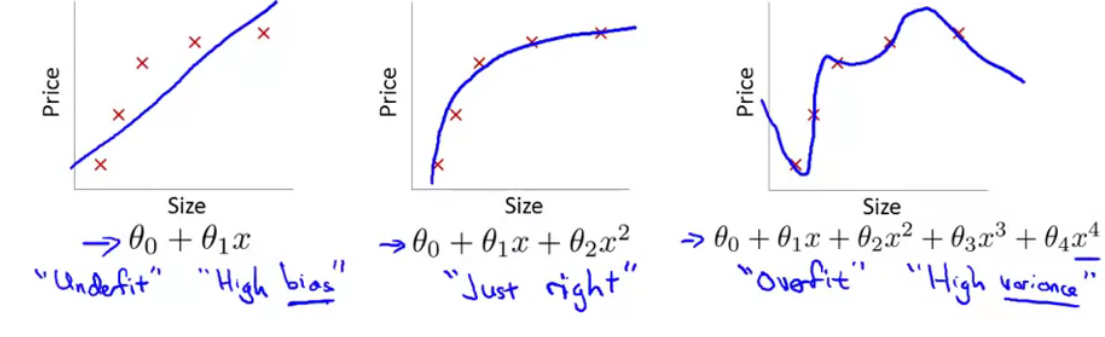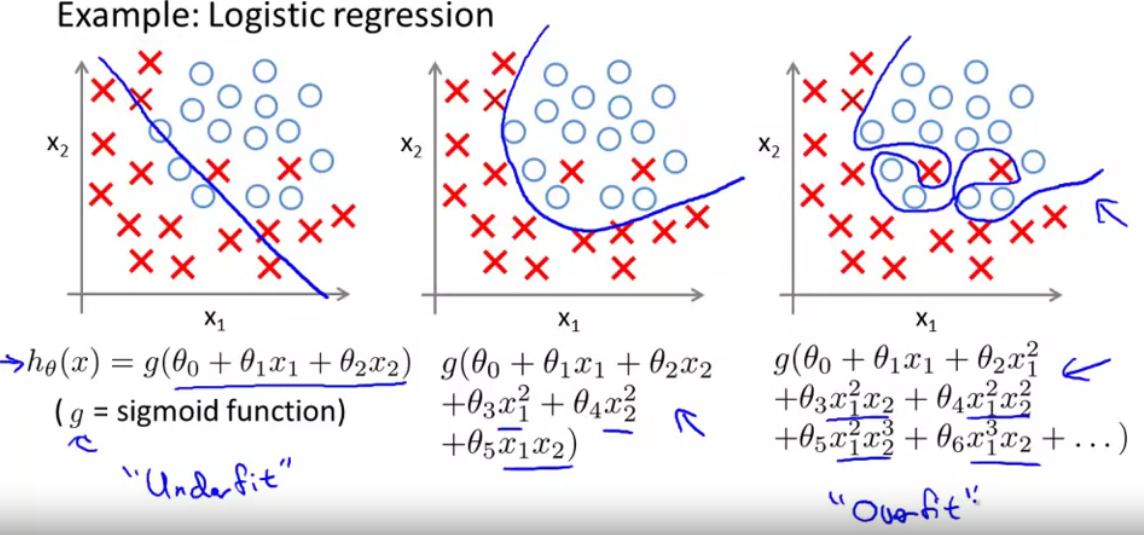## Types

• Using a model that is more flexible than it needs to be.

For example, a neural net is able to accommodate some curvilinear relationships and so is more flexible than a simple linear regression. But if it is used on a data set that conforms to the linear model, it will add a level of complexity without any corresponding benefit in performance or, even worse, with poorer performance than the simpler model.

• Using a model that includes irrelevant components

For example a polynomial of excessive degree or a multiple linear regression that has irrelevant as well as the needed predictors.

## Drawback

• Adding predictors that perform no useful function - waste resources but expands possibilities for undetected errors
• In feature selection problem, models that include unneeded predictors lead to worse decisions.
• Make predictions worse because of adding random variation
• Make your model not portable

## Solutions

• Reduce the number of features: manually select which features to be eliminated, a select model selection algorithm
• Regularization: Keep all the features, but reduce the magnitude of values of parameters $\theta_j$

2.Drive your weights to lower values

• Dropout: During training, drop out samples from an exponential number of different “thinned” networks. By dropping a unit out, we mean temporarily removing it from the network, along with all its incoming and outgoing connections. The choice of which units to drop is random.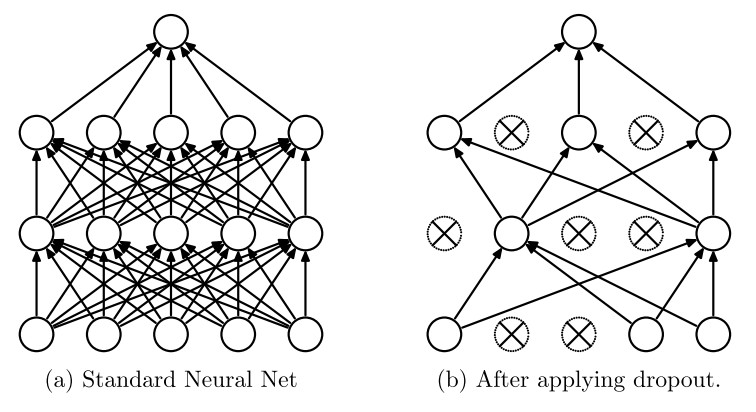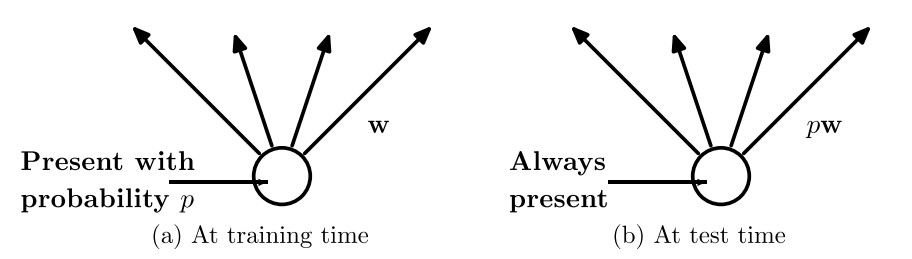# Class review

## Questions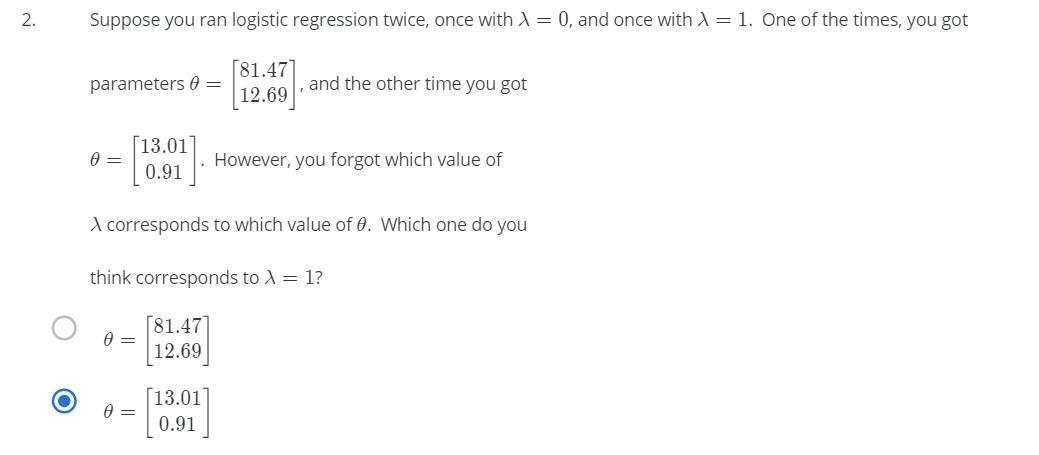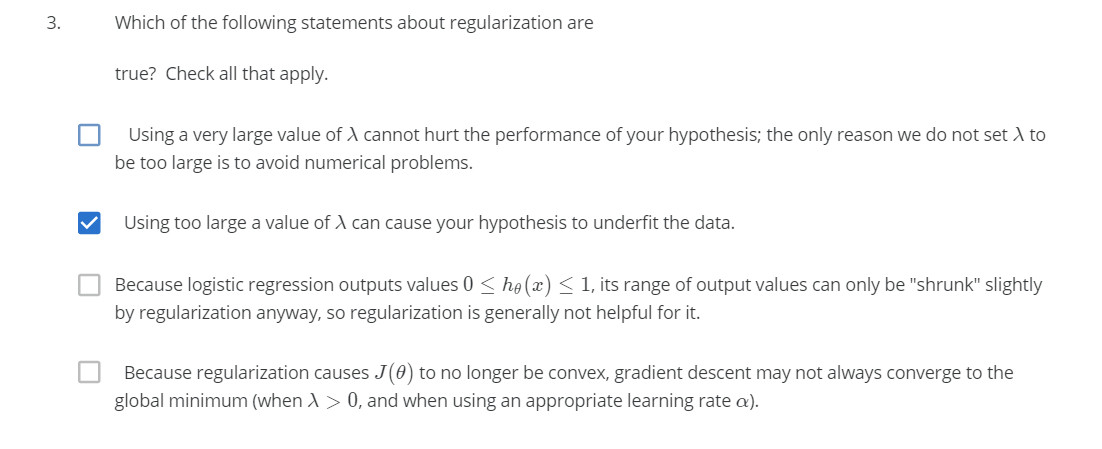## Others

Data normalization: $\frac{x - \mu}{\sigma}$

For logistic regression, you want to optimize the cost function $J(\theta)$ with parameters. Concretely, you are going to use fminunc() to find the best parameters $\theta$ for the logistic regression cost function, given a fixed dataset (of X and y values). You will pass to

fminunc the following inputs:
With a larger $\lambda$, you should see a plot that shows a simpler decision boundary which still separates the positives and negatives fairly well. However, if $\lambda$ is set to too high a value, you will not get a good fit and the decision boundary will not follow the data so well, thus underfitting the data (Figure 6).

# Reference

 Hawkins, D.M., 2004. The problem of overfitting. Journal of chemical information and computer sciences, 44(1), pp.1-12.

 Srivastava, N., Hinton, G., Krizhevsky, A., Sutskever, I. and Salakhutdinov, R., 2014. Dropout: a simple way to prevent neural networks from overfitting. The journal of machine learning research, 15(1), pp.1929-1958.

 Andrew NG, Machine Learning